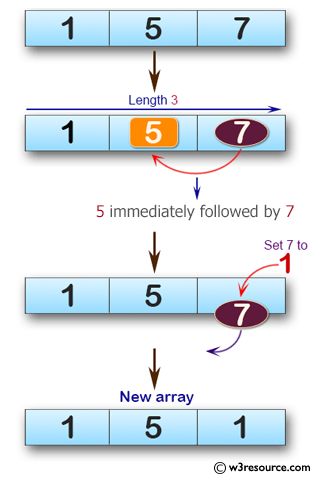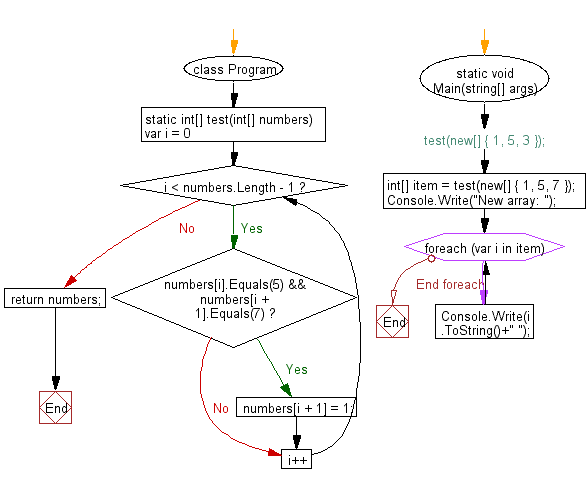﻿ C# - Array of integers with length 3 and create a new array# C# Sharp Basic Algorithm Exercises: Check a given array of integers, length 3 and create a new array

## C# Sharp Basic Algorithm: Exercise-101 with Solution

Write a C# Sharp program to check a given array of integers, length 3 and create a new array. If there is a 5 in the given array immediately followed by a 7 then set 7 to 1.

Pictorial Presentation:Sample Solution:-

C# Sharp Code:

``````using System;
namespace exercises
{
class Program
{
static void Main(string[] args)
{
//test(new[] { 1, 5, 3 });
int[] item = test(new[] { 1, 5, 7 });
Console.Write("New array: ");
foreach(var i in item)
{
Console.Write(i.ToString()+" ");
}
}
static int[] test(int[] numbers)
{
for (var i = 0; i < numbers.Length - 1; i++)
{
if (numbers[i].Equals(5) && numbers[i + 1].Equals(7))
numbers[i + 1] = 1;
}
return numbers;
}
}
}
```
```

Sample Output:

`New array: 1 5 1`

Flowchart:C# Sharp Code Editor:

Improve this sample solution and post your code through Disqus

What is the difficulty level of this exercise?

Test your Programming skills with w3resource's quiz.

﻿